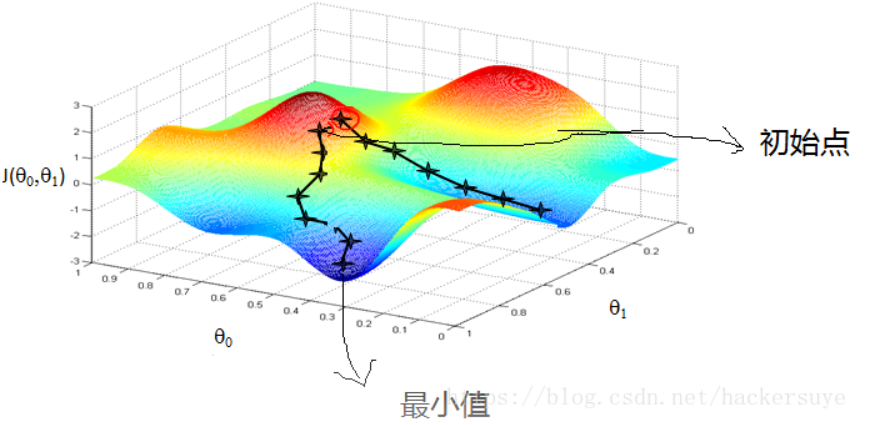• 泛函极值 变分法 梯度下降流1、积分泛函 欧拉-拉格朗日方程2、积分泛函 梯度下降流 在上一篇文章基于区域主动轮廓模型-Chan Vese模型中，我们通过构造能量泛函，从而巧妙把图像中目标分割问题转化为求解能量...
泛函极值 变分法 梯度下降流1、积分泛函 欧拉-拉格朗日方程2、积分泛函 梯度下降流3、以Chan-Vese模型为例的推导过程
在上一篇文章基于区域的主动轮廓模型-Chan Vese模型1中，我们通过构造能量泛函，从而巧妙的把图像中的目标分割问题转化为求解能量泛函极值的问题，然后利用变分法获得曲线演化方程。有人提出了这个推导原理或者推导过程是怎么回事，所以我今天专门写篇博客(参考重庆大学唐利明博士论文2 )分析以下这个问题。
1、积分泛函 欧拉-拉格朗日方程
假设能量泛函为: $E(u)=\int_{a}^{b} F\left(x, u, u^{\prime}\right) d x \tag{1}$我们的目标则是寻找目标函数$u(x)$使得能量函数$E(u)$取极小值，假设函数$u(x)$能够使得$E(u)$取极小值，那么对函数$u(x)$引入任意小的扰动后，或者说对于任意的$u(x)+\alpha v(x)$都有：$E(u) \leqslant E(u+\alpha v)$其中$\alpha$为常数，$v(a) =v(b)=0$，那么将$E(u+\alpha v)$看成是$\alpha$的函数$\phi(\alpha)$，那么对于一元函数$\phi(\alpha)$在$\alpha=0$的导数也就是泛函$E$的一阶变分$\delta E$应等于0，即$\delta E =\left.\frac{d \phi}{d \alpha}\right|_{\alpha=0}=\int_{a}^{b}\left(\frac{\partial F\left(x, u, u^{\prime}\right)}{\partial u} v+\frac{\partial F\left(x, u, u^{\prime}\right)}{\partial u^{\prime}} v^{\prime}\right) d x = 0$
进一步整理可得：$\delta E =\int_{a}^{b}\left(\frac{\partial F}{\partial u} v+\frac{\partial F}{\partial u^{\prime}} v^{\prime}\right) d x=0$，然后利用分步积分法对上式进一步分析推导：\begin{aligned} \delta E &=\int_{a}^{b}\left(\frac{\partial F}{\partial u} v+\frac{\partial F}{\partial u^{\prime}} v^{\prime}\right) d x=\int_{a}^{b} \frac{\partial F}{\partial u} v d x+\int_{a}^{b} \frac{\partial F}{\partial u^{\prime}} dv \\ & =\int_{a}^{b}\left[\frac{\partial F}{d u} v-\frac{d}{d x}\left(\frac{\partial F}{\partial u^{\prime}}\right) v\right] dx+\left.v \frac{\partial F}{\partial u^{\prime}}\right|_{a} ^{b}=0 \end{aligned}因为$v(a) = v(b) = 0$,那么$\delta E=\int_{a}^{b}\left[\frac{\partial F}{\partial u}-\frac{d}{d x}\left(\frac{\partial F}{\partial u^{\prime}}\right)\right] v d x=0$，如果对于任意函数$v$都能成立，那么根据变分法引理，$\frac{\partial F}{\partial u}-\frac{d}{d x}\left(\frac{\partial F}{\partial u^{\prime}}\right)=0$ 这个引理的证明也很简单，利用反证法，只需假设$v = (\frac{\partial F}{\partial u}-\frac{d}{d x}(\frac{\partial F}{\partial u^{\prime}}) )(x-a)(x-b)$，因为$(x-a)(x-b)<0$，所以$\delta E < 0$，与已知矛盾，故
$\frac{\partial F}{\partial u}-\frac{d}{d x}\left(\frac{\partial F}{\partial u^{\prime}}\right)=0 \tag{2}$ 上式就是大名鼎鼎的欧拉-拉格朗日方程(Euler-Lagrange),对于积分泛函内包含高阶导数的问题，比如$E(u)=\int_{a}^{b} F\left(x, u, u^{\prime}, u^{\prime \prime}\right) d x$利用上述变分法，完全可以获得对应的Euler-Lagrange方程
$\frac{\partial F}{\partial u}-\frac{d}{d x}\left(\frac{\partial F}{\partial u^{\prime}}\right)+\frac{d^2}{d x^2}\left(\frac{\partial F}{\partial u^{\prime \prime}}\right)=0 \tag{3}$对于变函积分内包括多元函数的问题，比如
$E(u)=\iint_{\Omega} F\left(x, y, u, u_{x}, u_{y}, u_{x}, u_{y y}\right) d x d y \tag{4}$同样可以推导出其对应的Euler-Lagrange
$\frac{\partial F}{d u}-\frac{d}{d x}\left(\frac{\partial F}{\partial u_{x}}\right)-\frac{d}{d y}\left(\frac{\partial F}{\partial u_{y}}\right)+\frac{d^{2}}{d x^{2}}\left(\frac{\partial F}{\partial u_{x x}}\right)+\frac{d^{2}}{d y^{2}}\left(\frac{\partial F}{\partial u_{y y}}\right)=0 \tag{5}$不过对于在大部分情况下，我们获得的Euler-Langarange方程一般都是非线性的偏微分方程，很难直接获得对应的解析解，因此，我们需要引入辅组的时间变量，将求解静态偏微分方程问题转化为动态偏微分方程问题，当演化至稳态时，就可以得到我们想要的偏微分方程的解了。
2、积分泛函 梯度下降流
假设随时间变化的函数$u(\cdot ,t)$能够使得$\delta E < 0$，那么能量泛函$E(u(\cdot, t))$则会不断减小，那么$u(\cdot ,t)$从时间$t$ 到时间$t + \Delta t$则会给能量函数引入一个新的扰动$V=\frac{\partial u}{\partial t} \Delta t$那么对应的
$\delta E=\int_{a}^{b}\left[\frac{\partial F}{\partial u}-\frac{d}{d x}\left(\frac{\partial F}{\partial u^{\prime}}\right)\right] v d x=\Delta t \int_{a}^{b}\left[\frac{\partial F}{\partial u}-\frac{d}{d x}\left(\frac{\partial F}{\partial u^{\prime}}\right)\right] \frac{\partial u}{\partial t} d x<0$
要想使上式恒成立，则可取(充分不必要但是最简洁)
$\frac{\partial u}{\partial t}=-\frac{\partial F}{\partial u}+\frac{d}{d x}\left(\frac{\partial F}{\partial u^{\prime}}\right) \tag{6}$
那么对于能量泛函$E(u)=\int_{a}^{b} F\left(x, u, u^{\prime}\right) d x$,想要获得其数值解，则需要构造偏微分方程\$$\left\{\begin{array}{l}\frac{\partial u}{\partial t}=\delta E(u) \\ u(x, 0)=u_{0}(x)\end{array}\right.$通过计算上述方程组，不断迭代计算，就能够找到使得能量函数$E(u)$取得极小值的函数$u(x)$。
3、以Chan-Vese模型为例的推导过程
关于Chan-Vese模型的详细内容大家可以看这一篇我的另一篇博文Chan-Vese模型。已知模型的能量泛函为：$E(\phi(x,y)) =\mu \int_\Omega{|\nabla H(\phi(x,y))|}dxdy + \nu \int_\Omega{H( \phi(x,y)}dxdy \\ + \lambda_1 \int_{\Omega}{|I_0(x,y)-C_1|^2 H( \phi(x,y)}\,{\rm d}x{\rm d}y + \\ \lambda_2 \int_{outside(C)}{|I_0(x,y)-C_2|^2 (1-H( \phi(x,y))}\,{\rm d}x{\rm d}y \tag{7}$ 那么怎样借助上面的梯度下降流公式来获得对应的用来演化的偏微方程：$\frac{\partial \phi}{\partial t} = \delta_{\epsilon}(\phi)[\mu div(\frac{\nabla\phi}{|\nabla\phi|})-\nu - \lambda_1(I-C_1)^2+\lambda_2(I-C_2)^2] \tag{8}$在开始推导之前我首先需要补充几点内容：
1.$div$是表示散度的意思，是一个标量值，比如说对于一个梯度向量$(f_x,f_y)$，则该梯度向量对应的散度值为$\frac{\partial}{\partial x}f_x + \frac{\partial}{\partial y}f_y$或者说$f_{xx}+f_{yy}$;
2. 公式(7)中的函数$H(\phi)$为Heaviside函数(也就是阶跃函数)，其对应的导函数为$\delta(\phi)$;
3. 公式(7)中的梯度的模值即$|\nabla H(\phi(x,y))|$的展开式为：$|\nabla H(\phi(x,y))| = \sqrt{\left(\delta(\phi) \phi_{x}\right)^{2}+\left(\delta(\phi) \phi_{y}\right)^{2}} = ({\left(\delta(\phi) \phi_{x}\right)^{2}+\left(\delta(\phi) \phi_{y}\right)^{2}}) ^{\frac{1}{2}}$
4. 公式(7)的能量函数中，$\phi(x,y)$是一个二元函数，且公式(7)中只存在$\phi(x,y)$的一阶导数，所以公式(7)对应的梯度下降流公式需要参照如下公式：$\frac{\partial u}{\partial t} = - \frac{\partial F}{\partial u}+\frac{d}{d x}\left(\frac{\partial F}{\partial u_x}\right)+\frac{d}{d y}\left(\frac{\partial F}{\partial u_y}\right) \tag{9}$
因此对于能量函数公式(7)，参照公式(9)，可得：
$\begin{array}{l} F: \mu|\nabla H(\phi)|+\nu H(\phi)+\lambda_{1}\left(I_{0}-C_{1}\right)^{2} H(\phi)+\lambda_{2}\left(I_{0}-C_{2}\right)^{2}(1-H(\phi)) \\ u: \phi \end{array}$进一步求导计算可得: $\frac{\partial F}{\partial \phi}=v \delta(\phi)+\lambda_{1}\left(I_{0}-C_{1}\right)^{2} \delta(\phi)-\lambda_{2}\left(I_{0}-C_{2}\right)^{2} \delta(\phi) \tag{10}$ 以及 \begin{aligned} \frac{d}{d x}\left(\frac{\partial F}{\partial \phi_{x}}\right)+\frac{d}{d y}\left(\frac{\partial F}{\partial \phi_{y}}\right) &=\mu\left(\frac{d}{d x}\left(\frac{\delta(\phi) \phi_{x}}{\sqrt{\left(\delta(\phi) \phi_{x}\right)^{2}+\left(\delta(\phi) \phi_{y}\right)^{2}}}\right)+\frac{d}{d y}\left(\frac{\delta(\phi) \phi_{y}}{\sqrt{\left(\delta(\phi) \phi_{x}\right)^{2}+\left(\delta(\phi) \phi_{y}\right)^{2}}}\right)\right) \\ &=\mu \delta(\phi) \operatorname{div}\left(\frac{\nabla \phi}{|\nabla \phi|}\right) \end{aligned} \tag{11}将公式(10)和公式(11)和整合进公式(9)后就可得到用来演化计算的偏微分方程：$\frac{\partial \phi}{\partial t} = \delta(\phi)[\mu div(\frac{\nabla\phi}{|\nabla\phi|})-\nu - \lambda_1(I-C_1)^2+\lambda_2(I-C_2)^2] \tag{12}$ 对上述计算或者推导过程有任何疑问或者觉得我没有表达清楚的，欢迎在评论区留言评论！！
参考文献

 Chan, T.F. and Vese, L.A., 2001. Active contours without edges. IEEE Transactions on image processing, 10(2), pp.266-277.

 唐利明.[基于变分方法的图像分解与图像分割][D]. 2013.


展开全文• 梯度下降算法是一种非常经典的求极小值算法，比如在线性回归里我们可以用最小二乘法去解析最优解，但是其中会涉及到对矩阵逆，由于多重共线性问题存在是很让人难受，无论进行L1正则化Lasso回归还是L2正则...
梯度下降1. 什么叫梯度？2. 梯度有什么用？3. 怎么走？
梯度下降算法是一种非常经典的求极小值的算法，比如在线性回归里我们可以用最小二乘法去解析最优解，但是其中会涉及到对矩阵求逆，由于多重共线性问题的存在是很让人难受的，无论进行L1正则化的Lasso回归还是L2正则化的岭回归，其实并不让人满意，因为它们的产生是为了修复此漏洞，而不是为了提升模型效果，甚至使模型效果下降。但是换一种思路，比如用梯度下降算法去优化线性回归的损失函数，完全就可以不用考虑多重共线性带来的问题。其实不仅是线性回归，逻辑回归同样是可以用梯度下降进行优化，因为这两个算法的损失函数都是严格意义上的凸函数，即存在全局唯一极小值，较小的学习率和足够的迭代次数，一定可以达到最小值附近，满足精度要求是完全没有问题的。并且随着特征数目的增多（列如100000），梯度下降的效率将远高于去解析标准方程的逆矩阵。神经网络中的后向传播算法其实就是在进行梯度下降，GDBT(梯度提升树)每增加一个弱学习器（CART回归树）,近似于进行一次梯度下降，因为每一棵回归树的目的都是去拟合此时损失函数的负梯度，这也可以说明为什么GDBT往往没XGBoost的效率高，因为它没办法拟合真正的负梯度，而Xgboost 的每增加的一个弱学习器是使得损失函数下降最快的解析解。总之梯度下降算法的用处十分广泛，我们有必要对它进行更加深入的理解。
1. 什么叫梯度？
偏导数组成的向量。
2. 梯度有什么用？
1）沿正梯度方向走函数值增大
2）沿负梯度方向走函数值减小
3. 怎么走？
w=w−ρ⋅∂L/∂w


展开全文机器学习
• 从接触深度学习开始，就不断...怎么求呢？就是求损失函数最小值（或者能找到最小值）对应系数，我们希望损失函数值往波谷方向走，那么怎么知道波谷方向呢，就是梯度下降方向 所以，只要对各个系数求偏导
从接触深度学习开始，就不断听到随机梯度下降这个名词。那么这到底是个什么东东呢？

随机梯度下降是用来求损失函数系数的最优解的，

什么是损失函数呢？损失函数就是你模型的预测值和真实值之间的差距

怎么求呢？就是求损失函数最小值（或者能找到的最小值）对应的系数，我们希望损失函数值往波谷的方向走，那么怎么知道波谷的方向呢，就是梯度下降的方向

所以，只要对各个系数求偏导，偏导最小，则为最优解。

更简洁明了的可以参考博客http://www.cnblogs.com/aijianiula/p/5173315.html

展开全文深度学习 机器学习 人工智能
• ## 梯度下降法及matlab代码详解实现

万次阅读 多人点赞 2019-08-04 16:01:27
梯度与方向导数的关系为：梯度的方向与取得最大方向导数值的方向一致，而梯度的模就是函数在该点的方向导数的最大值。梯度下降算法事实上是多维函数的在某一点收敛的极小值，可以用这个算法迭代出在哪个点收敛，也...
基本概念
梯度下降法又被称为最速下降法(Steepest descend method)，其理论基础是梯度的概念。梯度与方向导数的关系为：梯度的方向与取得最大方向导数值的方向一致，而梯度的模就是函数在该点的方向导数的最大值。梯度下降算法事实上是求多维函数的在某一点收敛的极小值，可以用这个算法迭代出在哪个点收敛，也是求最小二乘问题的一种方法。先在脑海中想象一下，你站在一座山上，怎么找到最快下山的方法，这时你当然会朝着最陡峭的方向前进，到达一个点后，再次朝着陡峭的方向下山，从而循环这些步骤，到达山脚。事实上，这也是梯度下降算法名字的由来，如图所示。matlab代码实现
梯度下降法的原理，本文不再描述，请参阅其它资料。
梯度下降法函数function [k ender]=steepest(f,x,e)，需要三个参数f、x和e,其中f为目标函数，x为初始点,e为终止误差。输出也为两个参数，k表示迭代的次数，ender表示找到的最低点。
steep.m
function [k ender]=steepest(f,x,e)
%梯度下降法,f为目标函数（两变量x1和x2），x为初始点,如[3;4]
syms x1 x2 m; %m为学习率
d=-[diff(f,x1);diff(f,x2)];  %分别求x1和x2的偏导数，即下降的方向
flag=1;  %循环标志
k=0; %迭代次数
while(flag)
d_temp=subs(d,x1,x(1));      %将起始点代入，求得当次下降x1梯度值
d_temp=subs(d_temp,x2,x(2)); %将起始点代入，求得当次下降x2梯度值
nor=norm(d_temp); %范数
if(nor>=e)
x_temp=x+m*d_temp;            %改变初始点x的值
f_temp=subs(f,x1,x_temp(1));  %将改变后的x1和x2代入目标函数
f_temp=subs(f_temp,x2,x_temp(2));
h=diff(f_temp,m);  %对m求导，找出最佳学习率
m_temp=solve(h);   %求方程，得到当次m
x=x+m_temp*d_temp; %更新起始点x
k=k+1;
else
flag=0;
end
end
ender=double(x);  %终点
end

调用示例
syms x1 x2;
f=(x1-2)^2+2*(x2-1)^2;
x=[1;3];
e=10^(-20);
[k ender]=steepest(f,x,e)

结果

k =

27

ender =

2
1


缺点
梯度下降法的缺点：
（1）靠近极小值时收敛速度减慢，如下图所示；
（2）直线搜索时可能会产生一些问题；
（3）可能会“之字形”地下降。


展开全文• 一、指导思想 　# 只针对线性回归中使用 算法最优模型功能：预测新样本对应值； ...怎么得到最优模型：出最优模型对应参数； 怎么求解最优模型参数：通过数学方法，...
• 现在在机器学习里面的梯度下降算法更多是根据训练数据集去找到一个合适拟合函数，那么怎么样才能找到一个拟合度高函数使之在测试集上有较好泛化能力，此时就需要定义一个拟合函数模型和一个损失函数，当损失...机器学习 python 算法 pycharm 数据挖掘
• a在用梯度下降法训练模型时候，有时候我们要在训练前大概了解一下参数，那么怎么进行调试呢。Bobo老师分享了一种方法，我分享给大家。  1、主要思想 这种思想主要是根据导数定义，其实梯度就是多元导数对单个...调试
• 各位进来老铁帮个忙。。帮我把最后自己写那个提升算法完善一下。。测试集该怎么测试准确率？？？ 大佬补充 from sklearn.datasets import load_iris # 用决策树作为基础模型 from sklearn.tree import ...机器学习 AdaBoost 随机森林
• 模型 假设空间适合当前问题和数据集函数关系。简单来说就是使用什么映射函数（X和Y之间关系F，以及参数） ...本质上就是计算机算法，怎么数学问题最优化解。正规方程还是梯度下降等等。统计学习
• EM（Expectation Maximum）应用： EM算法有很多应用，最广泛就是GMM混合高斯模型、聚类...　（2）M-Step：根据计算得到Q，出含有θ似然函数下界并最大化它，得到新参数θ 　重复（1）和（2）..数据挖掘 机器学习
• 虽然现在已经开源了很多深度学习框架（比如MxNet，Caffe等），训练一个模型变得非常简单，但是你对损失函数求梯度怎么求的了解吗？相信很多人不一定清楚。虽然网上资料很多，但是质量参差不齐，常常看得眼花...
• %Plots平滑模型的梯度阈值 Truncation=20;%代价函数截断阈值 P=4;%Plots模型参数 s=10;%Plos模型参数 D=50;%parameter for converting cost into compatibility exp(-C/D) iter=50;%belief propagation 算法迭代...matlab
• 预测时均值和方差怎么求？ CNN中BN BN是由Google于2015年提出，这是一个深度神经网络训练技巧，它不仅可以加快了模型收敛速度，而且更重要是在一定程度缓解了深层网络中“梯度弥散”问题，从而使得训练...
• 正确的取值初始化可以加快模型的收敛，不恰当的模型初始化可能引发梯度的消失或爆炸，最终导致模型无法训练。 一、模型初始化不恰当可能引起梯度消失或爆炸 我们使用右边的模型： ...
• 梯度与方向导数的关系为：梯度的方向与取得最大方向导数值的方向一致，而梯度的模就是函数在该点的方向导数的最大值。梯度下降算法事实上是多维函数的在某一点收敛的极小值，可以用这个算法迭代出在哪个点收敛，也...
• 大部分激活函数相当于起到类似于简单开关的作用，比如sigmoid relu，和阶跃函数都有点儿关系，当然了，也有的看上去不怎么像开关。 所以总体来说还是引入各种非线性，增加模型的表达...在求梯度的时候，如果x都...
• 转载自: https://blog.csdn.net/u014380165/article/details/79632950我们...虽然现在已经开源了很多深度学习框架（比如MxNet，Caffe等），训练一个模型变得非常简单，但是你对损失函数求梯度怎么求的了解...softmax softmax求导
• 一对多过拟合与正则化过拟合问题什么是过拟合(overfitting)问题如何解决过拟合问题正则化代价函数正则化思想线性回归正则化梯度下降方法正规方程Logistic回归正则化梯度下降方法怎么求决策边界 分类问题...
• 怎么找到合适模型 找到一条最合适能够拟合数据线，即一个线性或非线性方程，出其参数，模型即可知。接下来问题是，如何这个参数？ 随意给定几个参数，得到模型跟最佳模型肯定有一定误差，据此，...
• c 为了使网络执行BP算法，不能用传统一维搜索法每次迭代步长，而必须把步长更新规则预先赋予网络，这种方法将引起算法低效。 2、网络训练失败可能性较大，其原因有： a 从数学角度看，BP算法为一种...matlab
• 怎么求？ 导入基于误分类损失函数，利用梯度下降法对损失函数极小化，从而求得感知机模型。 文章目录感知机定义损失函数随机梯度下降感知机对偶形式总结代码实现 感知机定义 线性方程： 对应是特征...
• 当我们求解线性回归问题时引入梯度下降算法目的是，为了找到代价函数最小值，也就是代价函数曲线最低点，如图： 那么当对于某些线性回归问题，我们可不可以直接让代价函数对参数θ求的偏导等于0，来算出代价...机器学习 线性回归 正规方程
• 在台湾大学林轩田老师视频中，推导说，这个权值实际上貌似梯度下降，权值定义成1/2ln((1-ε)/ε)，实际上是有梯度下降，求梯度取最陡得到。 2、弱分类器怎么通常选啥 　可以选树（不选全树） 3、有了第一个弱...
• 毕竟我们在做梯度下降时候需要找到是最小损失值，损失值得方法都是错，再怎么搞都是南辕北辙。可以换一种简单点理解就是，损失函数得到损失值越小就表示我们预测值越接近真实值。 既然这么重要，那看看...损失函数 tensorflow 深度学习 神经网络